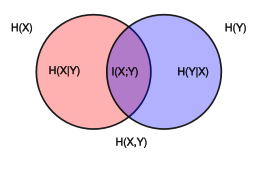# 信息论中的各种熵# 熵

H(X)=E[log2(X)]=p(x)log2(p(x))

# 联合熵

H(X,Y)=p(x,y)log(p(x,y))

# 条件熵

H(Y|X)=p(x,y)log(p(y|x))

H(X,Y)=H(X)+H(Y|X)=H(Y)+H(X|Y)=H(Y,X)

# 自信息

I(x)=log(p(x))

H(X)=E[log2(X)]=p(x)log2(p(x))

# 互信息

I(X;Y)=p(x,y)log(p(x)p(y)p(x,y))I(X;Y)=H(X)H(X|Y)=H(Y)H(Y|X)=H(X)+H(Y)H(X,Y)=H(X,Y)H(X|Y)H(Y|X)

# KL散度(信息增益)

KL散度，又称为相对熵（relative entropy）、信息散度（information divergence）、信息增益（information gain）。

KL散度是两个概率分布P和Q差别非对称性的度量。KL散度用来度量基于Q的编码来编码来自P的样本平均所需的额外的位元数。典型情况下，P表示数据的真实分布，Q表述数据的模型分布。

DKL(P||Q)=iP(i)logP(i)Q(i)

# 交叉熵

H(p,q)=Ep[logq]=H(p)+Dkl(p|q)

H(p,q)=p(x)log(q(x))

• qy=1=y^=g(wx)$q_{y=1} = \hat{y} = g(wx)$
• qy=0=1y^=1g(wx)$q_{y=0} = 1-\hat{y} = 1-g(wx)$

• py=1=y$p_{y=1} = y$
• py=0=1y$p_{y=0} = 1-y$

H(p,q)=p(x)logq(x)=ylog(y^)(1y)log(1y^)

L(w)=1Nn=1NH(pn,qn)=1Nn=1N[ylog(y^)(1y)log(1y^)]=1Nn=1Nlog(1+exp(ynwxn))09-065343

#### 关于熵的知识——信息论基本概念

12-07421

#### 信息论中的熵

09-28770

#### 信息论：熵与互信息

05-284万+

#### 信息论的熵

04-151913

#### 常见的各种熵

12-171631

#### 用c编写求信息论中各种熵和信息量的程序

12-017980

#### 信息论几个基本概念

07-101万+

#### 信息熵和图像熵

12-0628

#### 信息论——熵

10-191万+

#### 熵与信息增益

12-10324

#### 信息论模型-熵问题和互信量

09-18467

#### 信息论——信息熵

06-251074

#### 信息论笔记—熵

03-27889

#### 信息论——熵——信息熵

08-1552万+

#### Python+OpenCV计算机视觉

12-28©️2020 CSDN 皮肤主题: 大白 设计师: CSDN官方博客点击重新获取扫码支付1.余额是钱包充值的虚拟货币，按照1:1的比例进行支付金额的抵扣。
2.余额无法直接购买下载，可以购买VIP、C币套餐、付费专栏及课程。余额充值